# Cut Set and Cut Vertex of Graph

Whether it is possible to traverse a graph from one vertex to another is determined by how a graph is connected. Connectivity is a basic concept in Graph Theory. Connectivity defines whether a graph is connected or disconnected.

## Connectivity

A graph is said to be connected if there is a path between every pair of vertex. From every vertex to any other vertex, there should be some path to traverse. That is called the connectivity of a graph. A graph with multiple disconnected vertices and edges is said to be disconnected.

## Cut Vertex

Let 'G' be a connected graph. A vertex V ∈ G is called a cut vertex of 'G', if 'G-V' (Delete 'V' from 'G') results in a disconnected graph. Removing a cut vertex from a graph breaks it in to two or more graphs.

Note − Removing a cut vertex may render a graph disconnected.

A connected graph 'G' may have at most (n–2) cut vertices.

## Example

In the following graph, vertices 'e' and 'c' are the cut vertices.By removing 'e' or 'c', the graph will become a disconnected graph.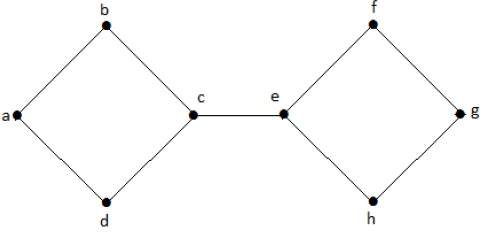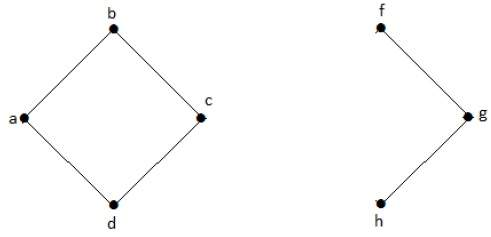Without 'g', there is no path between vertex 'c' and vertex 'h' and many other. Hence it is a disconnected graph with cut vertex as 'e'. Similarly, 'c' is also a cut vertex for the above graph.

## Cut Edge (Bridge)

Let 'G' be a connected graph. An edge 'e' ∈ G is called a cut edge if 'G-e' results in a disconnected graph.

If removing an edge in a graph results in to two or more graphs, then that edge is called a Cut Edge.

## Example

In the following graph, the cut edge is [(c, e)]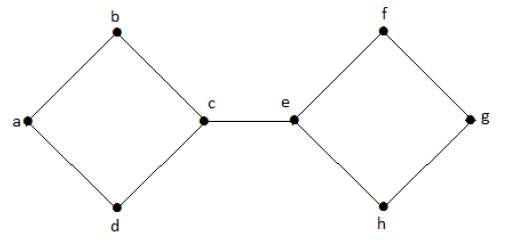By removing the edge (c, e) from the graph, it becomes a disconnected graph.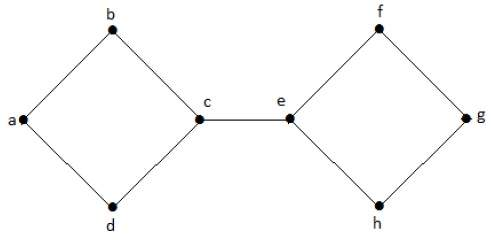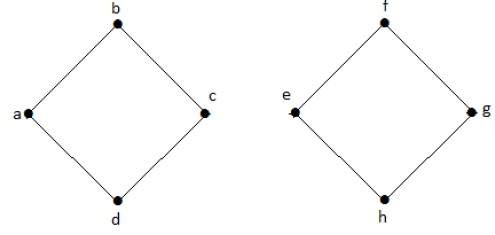In the above graph, removing the edge (c, e) breaks the graph into two which is nothing but a disconnected graph. Hence, the edge (c, e) is a cut edge of the graph.

Note − Let 'G' be a connected graph with 'n' vertices, then

• a cut edge e ∈ G if and only if the edge 'e' is not a part of any cycle in G.

• the maximum number of cut edges possible is 'n-1'.

• whenever cut edges exist, cut vertices also exist because at least one vertex of a cut edge is a cut vertex.

• if a cut vertex exists, then a cut edge may or may not exist.

## Cut Set of a Graph

Let 'G'= (V, E) be a connected graph. A subset E' of E is called a cut set of G if deletion of all the edges of E' from G makes G disconnect.

If deleting a certain number of edges from a graph makes it disconnected, then those deleted edges are called the cut set of the graph.

## Example

Take a look at the following graph. Its cut set is E1 = {e1, e3, e5, e8}.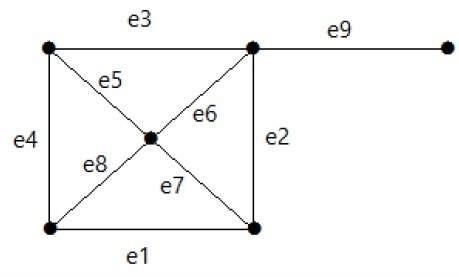After removing the cut set E1 from the graph, it would appear as follows −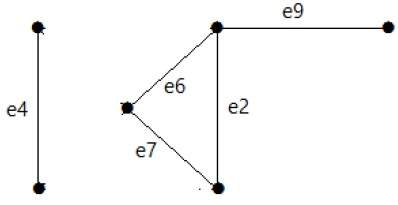Similarly there are other cut sets that can disconnect the graph −

• E3 = {e9} – Smallest cut set of the graph.
• E4 = {e3, e4, e5}

Updated on: 23-Aug-2019

17K+ Views